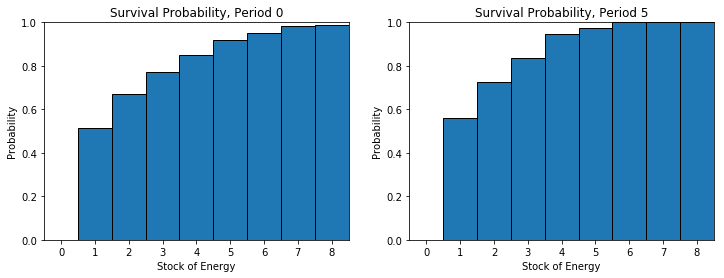# DiscreteDP Example: Bioeconomic Model¶

Daisuke Oyama

Faculty of Economics, University of Tokyo

From Miranda and Fackler, Applied Computational Economics and Finance, 2002, Section 7.6.6

In :
%matplotlib inline

In :
import numpy as np
import scipy.sparse as sp
import matplotlib.pyplot as plt
import quantecon as qe
from quantecon.markov import DiscreteDP, backward_induction, sa_indices

In :
emax = 8             # Energy carrying capacity
n = emax + 1         # Number of states, 0, ..., emax
m = 3                # Number of areas (actions), 0, ..., m-1
e = [2, 4, 5]        # Energy offerings
p = [1.0, 0.7, 0.8]  # Survival probabilities
q = [0.5, 0.8, 0.7]  # Success probabilities

T = 10               # Time horizon

# Terminal values
v_term = np.ones(n)
v_term= 0


We follow the state-action pairs formulation approach.

In :
L = n * m  # Number of feasible state-action pairs
s_indices, a_indices = sa_indices(n, m)

In :
# Reward vector
R = np.zeros(L)

In :
# Transition probability array
Q = sp.lil_matrix((L, n))
it = np.nditer((s_indices, a_indices), flags=['c_index'])
for s, k in it:
i = it.index
if s == 0:
Q[i, 0] = 1
elif s == 1:
Q[i, np.minimum(emax, s-1+e[k])] = p[k] * q[k]
Q[i, 0] = 1 - p[k] * q[k]
else:
Q[i, np.minimum(emax, s-1+e[k])] = p[k] * q[k]
Q[i, s-1] = p[k] * (1 - q[k])
Q[i, 0] = 1 - p[k]

In :
# Discount factor
beta = 1

In :
# Create a DiscreteDP
ddp = DiscreteDP(R, Q, beta, s_indices, a_indices)

//anaconda/lib/python3.6/site-packages/quantecon/markov/ddp.py:416: UserWarning: infinite horizon solution methods are disabled with beta=1
warnings.warn(msg)


Let us use the backward_induction routine to solve our finite-horizon problem.

In :
vs, sigmas = backward_induction(ddp, T, v_term)

In :
fig, axes = plt.subplots(1, 2, figsize=(12, 4))

ts = [0, 5]
for i, t in enumerate(ts):
axes[i].bar(np.arange(n), vs[t], align='center', width=1, edgecolor='k')
axes[i].set_xlim(0-0.5, emax+0.5)
axes[i].set_ylim(0, 1)
axes[i].set_xlabel('Stock of Energy')
axes[i].set_ylabel('Probability')
axes[i].set_title('Survival Probability, Period {t}'.format(t=t))

plt.show()In [ ]: